# Storage of variables in Apple-1 Integer BASIC

The purpose of this document is to clarify how variables are stored in RAM and how this information can be used. This information applies to Apple-1 (both Originals and Replicas) and to most of the emulators.

A brief recap, taken from the Apple-1 PRELIMINARY BASIC USERS MANUAL: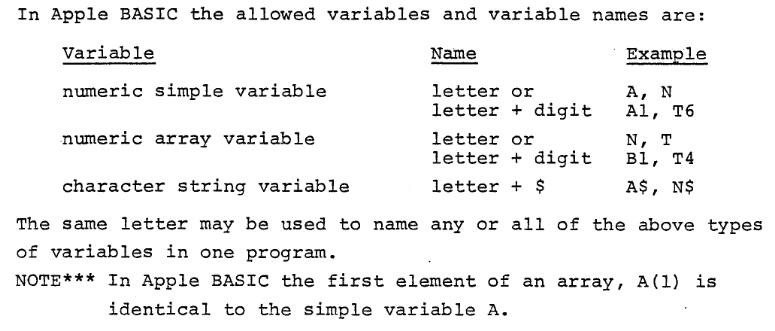Let us suppose, from now on, that the memory configuration is “standard” according to the indications in the manual:

• PRIMARY RAM BANK from address \$0000 to \$0FFF (4096 bytes)
• OPTIONAL RAM BANK from address \$E000 to \$EFFF (4096 bytes)

BASIC will be loaded in the optional RAM BANK.
We will not consider memory manipulation made by LOMEM or HIMEM commands.

When BASIC is running the user’s RAM, by default, is from \$0800 to \$0FFF (2047 bytes).

## 1. Program not using variables

A BASIC program is stored so that its end coincides with the last byte of memory available.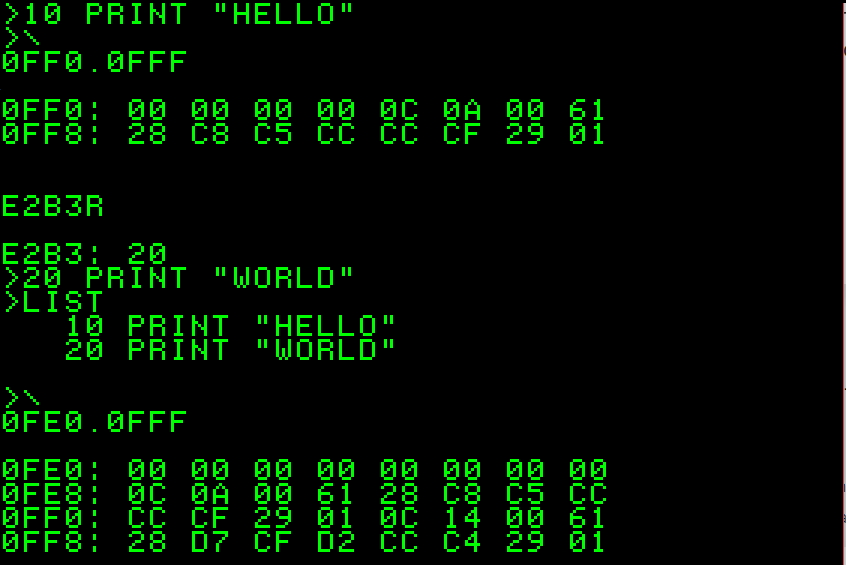In the two examples above, the one-liner 10 PRINT “HELLO” is stored from \$0FF4 to \$0FFF.
If a second line is added (20 PRINT “WORLD”) the entire program is “shifted”.
The two-line program now starts at \$0FE8 and ends at \$0FFF.

Is it reasonable to assume that variables will be stored at the opposite end of the available memory, in order to avoid any conflict with the program code. A quick inspection of the first locations shows nothing, which is also reasonable because our program uses no variables at all: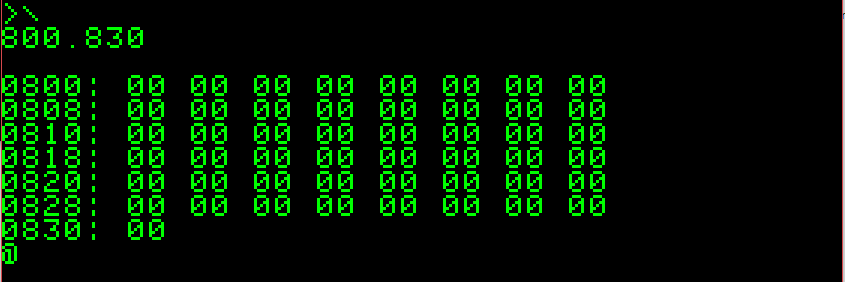## 2. Program using numeric variables

Let us restart everything and declare a variable, and see what happens: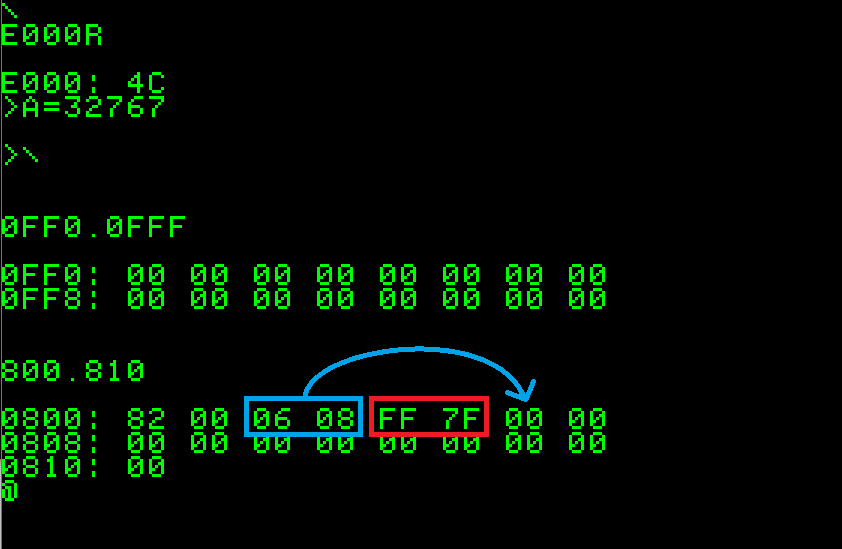As expected, nothing is stored in the “program area” of the memory, since we did not write any program.

I choose 32767 as value for the variable because it is hexadecimal \$7FFF and its HI and LO bytes are quite recognisable among all the others. They look to be stored in little-endian notation, two bytes as expected in locations \$0804 and \$0805. Are we able to spot out other informations from the hexdump? Sure we do: the couple of locations before the value appear to be the “end +1” of this variable declaration, in the usual little-endian notation: \$0806. It could also mean that address for the next variable is \$0806. How about \$82 and \$00 in location \$0800 and \$0801? We will get into the details in a little while, in the meantime: they are related to the variable name. ## 3. Program using more numeric variables Let us try now to declare two numeric variables and see what happens: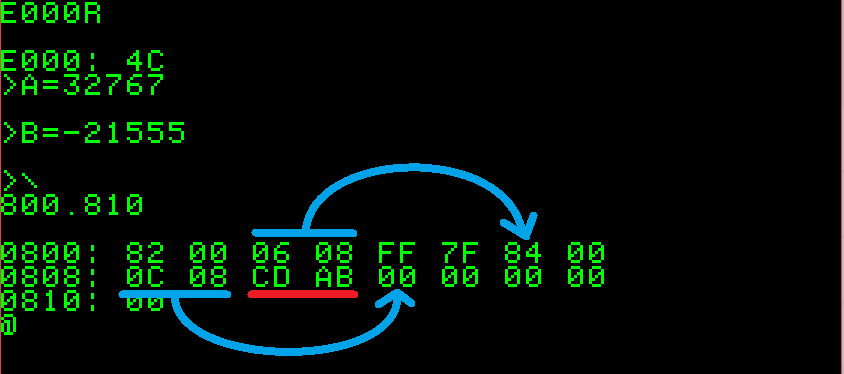Again, I chose a value for variable B easy to spot out: -21555, which is \$ABCD in signed integer INTEGER BASIC notation. \$CD and \$AB are clearly visible in the hexdump in the usual little-endian notation.

What else? Well, we can now see that the new variable starts where indicated from the previous one (\$0806), the first number is also \$84 instead of \$82. This could be something related to the variable name (“A” the first variable, “B” the second), while the next octet is still \$00.
After this, we can see the pointer to the next variable (\$080C, now free) and, marked in red, the value of variable B. So far, we have understood that numeric variables and their content are daisy-chained in order not to waste memory space. ## 4. Program using numeric variables and strings Let us see what happens if we add a previously DIMed string variable.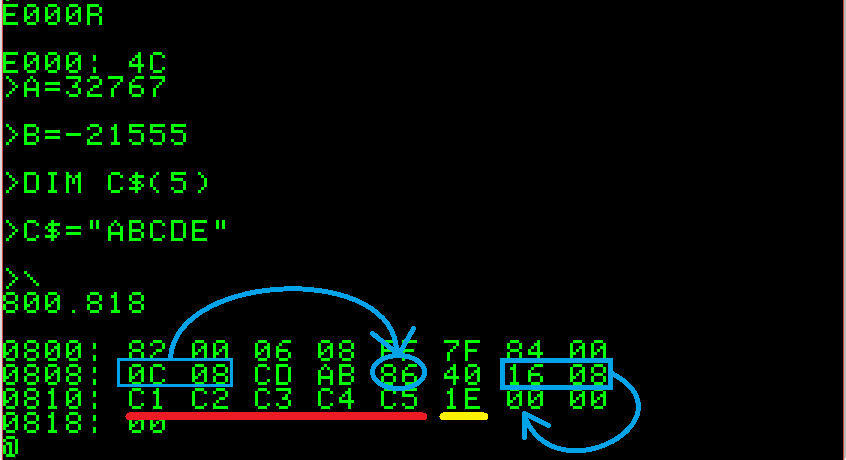Last variable we analysed, B, stated next variable address to be \$080C that was free.
Now at \$080C we find \$86.
This is even different from the \$82 used to define variable A and \$84 used to define variable B.
Curiously, \$86 is used to define variable C\$. We will see that this is no coincidence.
The next octet is \$40, which is different from \$00 we saw for integer variables.
This could mean that \$40 in the second position means “string”. Let’s see what’s next: \$16 and \$08 are, again, the pointer to the next variable: \$0816 (free, at the moment).
Then starts the content of the variable: \$C1, \$C2, \$C3, \$C4, \$C5 are the Apple-1 ASCII codes for ABCDE. Let’s keep in mind that Apple-1 ASCII has the MSB always set to 1: if standard ASCII hex for letter “A” is \$41 (01000001), Apple-1 will always assert MSB so binary will be 11000001, or hex \$C1. At the end of our string values, there is \$1E byte. Its meaning, among the ASCII non-printable characters is “Record Separator”. It is clearly an end-of-text marker and could be used by BASIC to retrieve the string content (one found among all the other) more quickly than analysing next variable pointer.

A non-DIMed variable, which can contain only one character, will have only one byte of RAM allocated, plus one for the \$1E Record Separator. It should also be clear now how a string is stored in RAM. The memory allocation take place only once the DIM command is issued, the content is written in the reserved space subsequently. ## 5. Program using also arrays How about arrays? Let’s have a look at the following example, keeping in mind that array can be only numeric: string arrays are not implemented.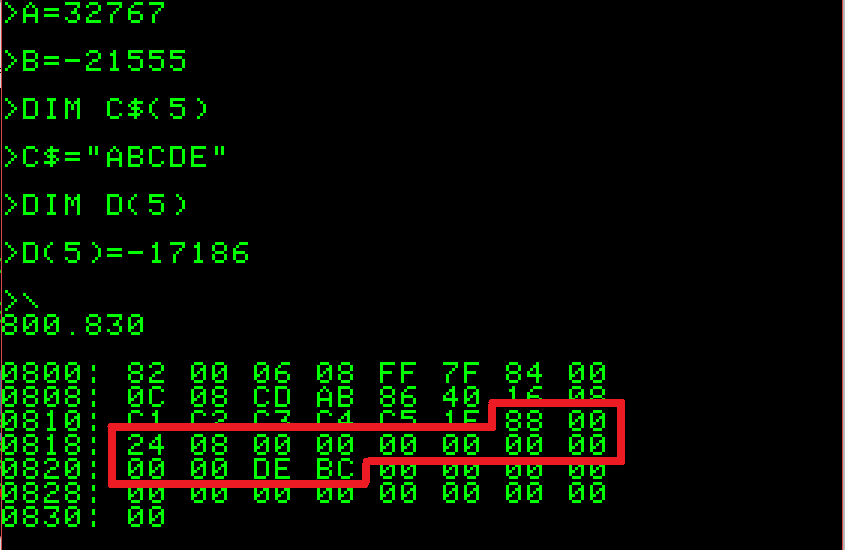A DIMensioning of the array D(5) has been added, and the value -17186 (\$BCDE) has been given to variable D(5), the last one.

I chose this value to highlight the boundary of the array with a recognisable couple of hexadecimal values.

In the picture above, we can clearly see that \$88 is the “name” of our array, \$00 means that is a numeric variable and \$0824 is the pointer to the next variable. Then follows a few bytes with no value, then the content of our array element D(5): \$BCDE in the usual format.
From this, we can tell that the DIMensioning of an array takes/reserves twice the bytes of the number of the elements of the array.
In addition: the uninitialized array elements are set to zero as stated in the BASIC Manual.
We can also tell that array elements 0 and 1 will have the same value, because we have only ten bytes allocated for our array of five elements.

The storage method of arrays is no different from what we saw in the previous examples.

## 6. Program using mixed letter + number variable name

We have only one last case of study, which is the mixed variable name: letter+number. This can be done only with numeric variables, no strings.

Let’s clear the emulator memory in order to reduce the number of informations displayed and try the following example:Let’s take for granted that we already know how numeric variables are stored and how pointer to next variable works. Now focus on the first and second octet of each variable, marked in yellow in the picture before.

We have:

\$82 \$00   for variable A
\$82 \$B1   for variable A1
\$82 \$B9   for variable A9

It is clear that second octet is the numeric part of the mixed-name variable. Until now, we have seen the second byte only set to \$00 for numeric variables and to \$40 for string variables, we now know that it can also assume values from \$B1 to \$B9.

Why use \$B1\$B9 value instead of 09?
The answer is, again, in the forcing to 1 of the MSB applied in the Apple-1 architecture.
ASCII 1 is hexadecimal \$31, which is binary 00110001. Set MSB to 1 lead to 10110001 or hexadecimal \$B1.
ASCII 2 is hexadecimal \$32, which is binary 00110010. Set MSB to 1 lead to 10110010 or hexadecimal \$B2.

And so on, so probably the numeric part of the mixed-name variable is taken as ASCII character.
The same applies also to numeric arrays with a composite name, i.e. DIM P5(10).
The second byte of the variable name will be set accordingly.

Before a final recap, let’s try to explain why variable name “A” is stored as \$82, “B” as \$84, “C” as \$86 and so on.  ASCII A is hexadecimal \$41, which is binary: 01000001 Hexadecimal \$82 is: 10000010 ASCII B is hexadecimal \$42, which is binary: 01000010 Hexadecimal \$84 is: 10000100 It is clear that the hexadecimal value used to store the variable name in RAM is the corresponding ASCII valued rotated (or shifted) left by one position. Because we are using a subset of the ASCII set, (just the letters A…Z) we can also assume that the value used is the ASCII value multiplied by two. It is not clear to me the reason for this special handling, certainly the condition “= \$82” cannot be used to scan the memory area in search of the variable A: \$82 could be a legitimate value of a numeric variable… but probably I am missing something. ## 7. Final notes A couple of final notes: if a LOMEM command is issued as first command, all the variables will be stored starting from the LOMEM address. If the declaration of variables is done inside a BASIC program, the memory will be allocated when the corresponding program line is executed. Therefore, this grid should recap how variables are stored on to the RAM of the Apple-1, just keep in mind to start at the beginning of the RAM area:Enjoy! ? April 2020, Claudio Parmigiani ## 8 – Sample program (by Francesco Sblendorio) 0 A$="X": REM BUFFER VARIABLE
10 DIM X(4):X(1)=873:X(2)=42:X(4)=90
20 DIM D9(3):D9(1)=700:D9(2)=18000:D9(3)=17
30 DIM C$(10): C$="TEST-1"
40 DIM R$(10): R$="FRANCO"
50 G1=150:G7=901
10000 I=2048
10010 GOSUB 20000:N=V/2+128:POKE 2052, N:PRINT A$; 10020 GOSUB 20000:T=V 10030 GOSUB 20000:N=V:GOSUB 20000:P=N+V*256 10040 IF T=64 THEN 11000 10050 IF T<>0 THEN PRINT T-176; 10060 L = P-I: Z = L 10070 IF (Z>2) THEN PRINT"(";(Z-L)/2+1;")"; 10080 GOSUB 20000:N=V:GOSUB 20000: PRINT " = ";N+V*256;: REM PRINT " [";N;" ";V;"]"; 10090 PRINT 10100 L=L-2:IF L<=0 THEN 13000 10110 PRINT A$;:IF T<>0 THEN PRINT T-176;
10120 GOTO 10070
11000 T = I: PRINT"$= "; 11010 GOSUB 20000: IF V=30 THEN 12000 11020 POKE2052,V:PRINT A$;:GOTO11010
12000 PRINT " [";: FOR T=T TO I-2 : PRINT PEEK(T);: IF T<>I-2 THEN PRINT " ";
12010 NEXT T: PRINT "]"
13000 IF PEEK(P)=0 THEN 19999
13010 I=P:GOTO 10010
19999 END
20000 V=PEEK(I):I=I+1:RETURN


### Step-by-step description

Line 0: Variable A$is a “buffer”. It’s very important that it’s the very first variable declared in the program: so we know exactly the address where is contained its content (2052 = \$0804). In this way we can read and write into it by POKing and PEEKing ASCII value. In particular, it will be used in lines 10010, 10093 and 11020 to “mimic” missing CHR$function (by POKing into 2052). Line 10-50: Sample variables that will be inspected by the rest of the program. Line 20000: Subroutine reading memory increasing a counter. Variable “I” is the current location to be read, variable “V” is the value read. Line 10000: Initializes couter to 2048 = \$0800, which is the start address for variables. It will be increased by subroutine in 20000

Line 10010: Reads and print first charcter of variable name

Line 10020: Reads type (T) of variable (64=string, 0=numeric, 177..185=numeric with second character in name)

Line 10030: Reads pointer (P) to next variable

Line 10040, 11000..12010: Prints name and content (ASCII and DEC) of a string variable.

Line 10050..10120: Print name and content (both single values and arrays) of numeric variable.

Line 13000..13010: Skip to next variable in memory, till \\$00 is found.

## Screenshot: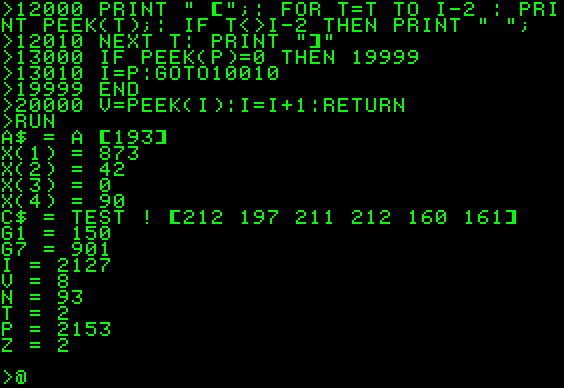## Bibliography:

Apple-1 Preliminary Apple Basic Users Manual
https://www.applefritter.com/files/basicman.pdf

Apple-1 Operation Manual
https://www.applefritter.com/files/a1man.pdf

Pom1 (Apple-1 Emulator, works with Windows / Android / Linux+wine)
http://pom1.sourceforge.net/